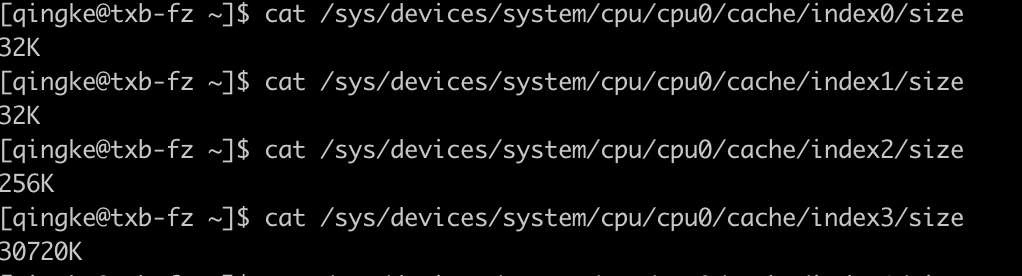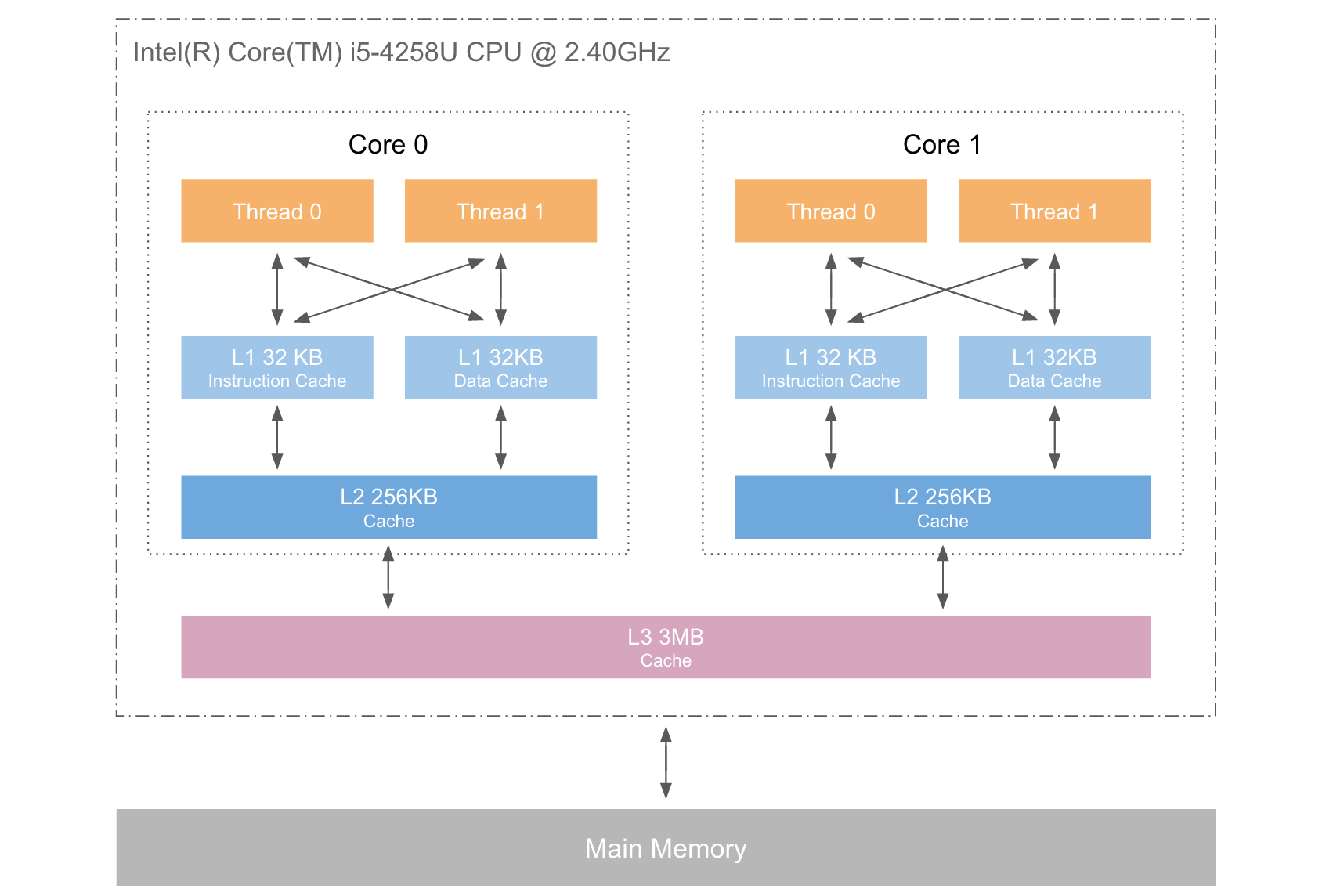# 啥是 CPU 缓存？又如何提高缓存命中率呢？# 1 什么是CPU缓存

### 1.1 CPU缓存的来历

CPU缓存的容量比内存小的多但是交换速度却比内存要快得多。缓存的出现主要是为了解决CPU运算速度与内存读写速度不匹配的矛盾，因为CPU运算速度要比内存读写速度快很多，这样会使CPU花费很长时间等待数据到来或把数据写入内存。所以，为了解决CPU运算速度与内存读写速度不匹配的矛盾，就出现了CPU缓存。

### 1.2 CPU缓存的概念

CPU缓存是位于CPU与内存之间的临时数据交换器，它的容量比内存小的多但是交换速度却比内存要快得多。为了简化与内存之间的通信，高速缓存控制器是针对数据块，而不是字节进行操作的。高速缓存其实就是一组称之为缓存行(Cache Line)的固定大小的数据块组成的，典型的一行是64字节。

### 1.3 CPU缓存的意义

CPU往往需要重复处理相同的数据、重复执行相同的指令，如果这部分数据、指令CPU能在CPU缓存中找到，CPU就不需要从内存或硬盘中再读取数据、指令，从而减少了整机的响应时间。所以，缓存的意义满足以下两种局部性原理

• 时间局部性（Temporal Locality）：如果一个信息项正在被访问，那么在近期它很可能还会被再次访问。

• 空间局部性（Spatial Locality）：如果一个存储器的位置被引用，那么将来他附近的位置也会被引用。

# 2 CPU的三级缓存

CPU 缓存离 CPU 核心更近，由于电子信号传输是需要时间的，所以离 CPU 核心越近，缓存的读写速度就越快。但 CPU 的空间很狭小，离 CPU 越近缓存大小受到的限制也越大。所以，综合硬件布局、性能等因素，CPU 缓存通常分为大小不等的三级缓存：L1L2L3。级别越小越接近CPU，所以速度也更快，同时也代表着容量越小。

L1 是最接近CPU的, 它容量最小（例如：32K），速度最快，每个核上都有一个 L1 缓存，L1 缓存每个核上其实有两个 L1 缓存, 一个用于存数据的 L1d Cache（Data Cache），一个用于存指令的 L1i Cache（Instruction Cache）。

L2 缓存 更大一些（例如：256K），速度要慢一些, 一般情况下每个核上都有一个独立的L2 缓存;

L3 缓存是三级缓存中最大的一级，同时也是最慢的一级, 在同一个CPU插槽之间的核共享一个 L3 缓存。如下这是我的开发机的CPU缓存大小情况。# 2 提升代码缓存命中率

## 2.1 GO基准测试

package arrayimport "testing"const LEN = 2048func BenchmarkArrayHead(t *testing.B) {	//连续访问	var arr [LEN][LEN]int	t.ResetTimer()	for i := 0; i < LEN; i++ {		for j := 0; j < LEN; j++ {			arr[i][j] = 0		}	}}func BenchmarkEnd(t *testing.B) {	//非连续访问	var arr [LEN][LEN]int	t.ResetTimer()	for i := 0; i < LEN; i++ {		for j := 0; j < LEN; j++ {			arr[j][i] = 0		}	}}

goos: darwingoarch: amd64pkg: demo/arrayBenchmarkArrayHead-8    1000000000               0.00372 ns/opBenchmarkEnd-8          1000000000               0.0240 ns/opPASSok      demo/array      0.279s

﻿

## 2.2 PHP基准测试

<?phpini_set('memory_limit', '256M');//初始化Packed Array$max = 2048;$arr = [];for ($i = 0;$i < $max;$i++) {    for ($j = 0;$j < $max;$j++) {        $arr[$i][$j] = 0; }}//非连续访问$stime = microtime(true);for ($i = 0;$i < $max;$i++) {    for ($j = 0;$j < $max;$j++) {        $arr[$j][$i] = 0; }}$etime = microtime(true);echo "非连续访问 :\t" . ($etime -$stime) . "\n";//连续访问$stime = microtime(true);for ($i = 0; $i <$max; $i++) { for ($j = 0; $j <$max; $j++) {$arr[$i][$j] = 0;    }}$etime = microtime(true);echo "连续访问 :\t" . ($etime - \$stime) . "\n";

﻿

 php test.php非连续访问 :    1.3713099956512连续访问 :      0.97811102867126

# 3 结论

﻿## 评论